### Expected Number of Attempts - Broken Coffee Machine

Related Problem: Expected Length of Last Straw - Breaking the back of a Camel - CSE Blog

Problem:

Your boss tells you to bring him a cup of coffee from the company vending machine. The problem is the machine is broken. When you press the button for a drink, it will randomly fill a percentage of the cup (between 0 and 100 percent). You know you need to bring a full cup back to your boss.

What’s the expected number of times you will have to fill the cup?

Example: The machine fills the cup 10 percent, then 30 percent, then 80 percent–>the cup is full plus 20 percent that you throw away or drink yourself. It took 3 fills of the cup.

1.2.7 or 3 times

2.btw we fill the cup only once...we press the button 3 times!

3.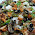x_i : The fraction of cup , the coffee machine fills in ith filling
This is uniformly distributed variable varying from 0 to 1

We would fill for i+1 th time if x1+x2+..x_i < 1
P(x1+x2...x_n < 1) = 1/n!
So
answer = 1 + P(x1<1) + P(x1+x2<1) + P(x1+x2+x3<1)....
= 1 + 1/1! + 1/2! + 1/3! ....
= e

1.Can you explain the logic behind this - P(x1+x2...x_n < 1) = 1/n! ?

2.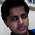Q(v,k) = Prob. of x1+x2+...xk adding < v for 0<=v<1
P.T. Q(v,k) = v^k/k!.
Proof by induction. True for k=1
Q(v,k+1) can be defined as:
for every value x of k+1'th variable, integration over probability that last k vars add up to (v-x)
=> Q(v,k+1) = Int(0->v)[Q(v-x,k).dx] = Int(0->v)[(v-x)^k/k!.dx] = v^(k+1)/(k+1)!
Hence proved by induction.
The value to be proved it Q(1,n) = 1/n!

3.say (i+1)th is time when its fill cup but is probability of filling at (i+1)th time is a sure event? i mean probability will be p(x1+x2+..x_i < 1)*(probability of filling at i+1 th time). time multiplied term is not 1 always.....

4.The integration result is wrong. The formula does not work!.

4.Using continuous probability. Let E(x)=expected number of trips required to fill up the cup upto x-th fraction.
Then E(x)=Integral fo (E(x-i)*(1-i)di) with i=0 to x,
We obtain at this integral by considering that first we need to fill upto a fraction x-i and then the machine a fraction greater than i the probability of which is 1-i.
Using substitution x=1-t, we obtain E(x)= Integral of (E(t)dt) with t from 0 to x.
Using second Fundamental theorem of calculus. dE(x)/dx=E(x), Integration with limits from x=0 to 1 and the boundary condition that E(0)=1, we get E(1)=e.

1.@Kushal: Why E(0)=1? Shouldn't the expected number of trips to fill the cup up to 0th fraction (i.e empty) be 0?

2.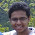You are right. The boundary condition at 0 is arguable. You can instead say that (lim x->0) E(x) = 1. Also, letting E(x) = c*e^x, the constant c can be found by substituting in the original equation, which by the way has some error. (Refer to the corrected solution in the comment below.)

5.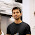Let f(x) denote the expected time to fill a glass with x liters. We can model this as a Markov chain and find the expected time to reach an absorbing state.

6.Let f(x) be the expected number of fills required to fill the glass upto (>x) fraction (DEFINED ON x<=1). Consider 2 cases on the fraction y of the next fill. If (y(y-x)) fraction more, having already filled once and thus, EXPECT to take 1 + f(x-y) fills in total. If (y>x), we just needed 1 fill. Thus, f(x) = integral [y=0 to x] of (1 + f(x-y)) dy + integral [y=x to 1] of 1 dy. Differentiating this with respect to x, we have: f'(x)= f(x) which gives f(x) = c.e^x; c can be evaluated to be 1, by substituting in the original equation. Thus, the answer is f(1) = e.

7.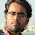Let the fraction of the cup of coffee that gets filled at any i_th fill be denoted by a random variable X which is given to be uniformly distributed between [0,1].
Now, Let S(n) denote the sum of these n uniform random variables.
The Cumulative distribution function of this random variable S(n) i.e. Pr(S(n) < x) =x/n!. For details, see https://en.wikipedia.org/wiki/Irwin%E2%80%93Hall_distribution.

The probability of the event that the cup gets filled at the nth fill conditioned on the event that in the nth fill, fraction x of cup is provided by the machine is.
Pr{ S(n) >= 1 and at nth fill 'x' fraction of cup gets filled } = Pr { S(n-1) >= 1-x && S(n-1) < 1 | at nth fill 'x' fraction of cup gets filled }
Clearly, the above event i.e. S(n-1) >= 1-x && S(n-1) < 1 ensures that cup gets filled in the nth fill given that the nth fill provides us with x fraction of cup of coffee.
Pr{ S(n-1) >= 1-x && S(n-1) < 1 } = {1 - (1-x)^(n-1)} / { (n-1) ! } (Using CDF of sum of uniform random variables)
Pr{ at nth fill 'x' fraction of cup gets filled } = pdf{X = x}dx. This is the probability that the fraction of the cup that gets filled is within x to x+dx
To get the total probability that Pr {S(n) >= 1}, we need to integrate over all fractions 'x' from 0 to 1.
Pr{ S(n) >= 1 } = integral[0,1] of Pr{ 1-x <= S(n-1) <= 1 }*(1) dx since pdf {X=x} = 1. (Uniform distribution).
Pr{ 1-x <= S(n-1) <= 1 } = (1 - (1-x)^(n-1) ) / ( (n-1) ! )
Pr{ S(n) >= 1 } = (1/n(n-2)! )
Its not hard to check Summation { n=2 to inf } (1/n(n-2)! ) = 1.
Therefore, expected no of fills required = Summation {n=2 to inf } 1/(n-2)! = e.

8.Great post!!, one quick question. You have mentioned that Pr(S(n) < x) =x/n!. Shouldn't it be Pr(S(n) < x) =x^n/n!

1.Sorry @Aashish Kumar, it's a typo. You are right Pr(S(n) < x) =x^n/n!. Thanks for pointing it out !

9.An interesting thing to note is that E[% full after pushing the button twice)]=100

10.Increadable. I expected in to be 2 because automata is expected to dispense 1/3 of cup in average. Yet, simulation http://scastie.org/12772 says that 2.7 is right!

11.The amount of coffee filled currently should not depend on how much coffee previously filled in the cup. So Markov model may not be a good option. I think 2 is correct answer since expected amount in one fill is 50%.